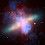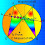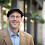## Tuesday, January 28, 2014

### Geometry Problem 969: Equilateral Triangle, Rectangle, Common Vertex, Right Triangles Areas

Geometry Problem
Level: Mathematics Education, High School, Honors Geometry, College.

Click the figure below to view the complete problem 969.1.Let z(P) be the complex number representing P.
Let z(C)=0, z(B)=−a, z(E)=−a−bi.

Then
z(F) = (−a−bi)(1/2 + √3/2 i) = (−1/2 a + √3/2 b) + (−√3/2 a − 1/2 b)i
z(D) = (−√3/2 a − 1/2 b)i
z(A) = −a + (−√3/2 a − 1/2 b)i

Thus
BC = a
BE = b
AE = √3/2 a − 1/2 b
DF = 1/2 a − √3/2 b
AF = 1/2 a + √3/2 b
CD = √3/2 a + 1/2 b

S₁ = 1/2 × AE × AF = √3/8 (a^2 − b^2) + 1/4 ab
S₂ = 1/2 × BC × BE = 1/2 ab
S₃ = 1/2 × CD × DF = √3/8 (a^2 − b^2) − 1/4 ab

Hence,
S₁ = S₂ + S₃

2.Use the area formula of triangle:
A=c^2/(2[cotA+cotB])

In this case, c^2/2 cancel out of the equation S2+S3=S1. What we are left with is a simple trigonometric identity which is always true.

3.Please if you want explain it more.
than you.

4.using trigonometry should always be the last resort. There must be another, direct, proof, using geometric reasoning.

5.Fie E(0,e),F(f,o)atunci C(f/2+e√3/2; e/2+f√3/2)pentru a arata ca S₁ = S₂ + S₃ trebuie sa aratam ca

6.Think about pythagoras triangle BEC and DFC

7.i think their might be wrong in the question'

8.Triangles EBC and FDC are congruent (RHS congruency)
=> BE = FD = x let, AE = AF = a − x where a is length of the side of square ABCD.
By Pythagoras Theorem, S2 + S3 = ax/2 + ax/2 = ax
S1 = (1/2)(a − x)^2

Now notice that EF = CF = CE
so (a − x)^2 + (a − x)^2 = a^2 + x^2
so (a − x)^2 = 2ax
so (1/2)(a − x)^2 = ax
=> S1 = S2 + S3

1.To Abdul Problem 969:
Triangles EBC and FDC are congruent (RHS congruency)
Thanks

9.BC = CD, CE = CF, m(EBC) = 90° = m(FDC)

1.To Abdul: In your statement BC = CD
ABCD is a rectangle (not necessary a square) therefore BC is not equal CD
Thanks.

10.Is there a simple geometric proof for this Antonio?

11.Let M be midpoint of EC so that AEMF and CDFM are cyclic quadrilaterals from which we can easily show that ADM is equilateral

Let BE = a, AE = b, AF = c, FD = d and FE = x

Apply Ptolemy to the cyclic quad AEMF we have

Sqrt3/2 xb + x/2 c = x(c+d) from which

b = (c+2d)/sqrt3.....(1)

Similarly from cyclic quad CDFM we have upon using Ptolemy and simplifying as before

a+b = (2c+d)/sqrt3 from which using (1) we have

a =(c-d)/sqrt3......(2)

So S 2 + S3 = 1/2a(c+d) + 1/2(a+b)d which upon substituting for a and b from (1) and (2)

= (1/2sqrt3){(c-d)(c+d) + (2c+d)d} = (1/2sqrt3)(c+2d)c = bc/2 = S1

Sumith Peiris
Moratuwa
Sri Lanka

12.https://goo.gl/photos/VRMyqEUhTyU6DB8e6

Draw equilateral triangle PDC
Let PE cut BC at F ( see sketch)
Triangle CDF congruent to CPE ( case SAS)
Triangles CPF and EBF are 30-60-90 triangles
With simple algebra calculation we have
EB= 2a-b.sqrt(3)
FD=EP=2b-ạsqrt(3)
And S2= ½.b(2a-b.sqrt(3))
S3= 1/2a(2b-ạsqrt(3))
S1= ½(b. sqrt(3)-a).(a .sqrt(3)-b)
Verify that S1=S2+S3

13.Angle chase and you have (x,90-x,90) (30+x,60-x,90) and (30-x, 60+x, 90) triangles.

Take them and line them all up to share the same hypotenuse. such that the that
C is the left corner of BCE, F is the left corner of CDF and E is the left corner of AEF,

Angle chase again and you'll now find six 30-60-90 triangles!

That's enough to get formulas for all three just in terms of BE and DF working through the sides.

See: https://pbs.twimg.com/media/DGXjr2YUQAAZPcv.jpg

From there its fairly simple to verify the equality.

14.Triangles EBC and CFD are congruent . Draw S2 sidewards S1
See sketch for explanation
https://photos.app.goo.gl/JBqEyJmzAVfuJ3Yb6

1.ABCD is a rectangle not a square. So EBC and CFD are not necessarily congruent